# Python in Neuroscience¶

## by Gilles de Hollander, March 2014¶

In this IPython Notebook I will demonstrate a large number of Python packages which are relevant for research in cognitive neuroscience.

# Basic Python¶

Python variables are somewhere in between dynamically and static typed variables: you don't have to give a type in advance:

In :
a = 2
b = '3'

a, b, type(a), type(b)

Out:
(2, '3', int, str)

But there is no implicit typecasting if it would be unclear what is the 'right' conversion. Here the code is ambivalent: do you want to cast a to a string to get '23' or do you want to cast b to an integer and get 5? Python does not know and gives an error...

In :
a+b

---------------------------------------------------------------------------
TypeError                                 Traceback (most recent call last)
<ipython-input-22-f1d53b280433> in <module>()
----> 1 a+b

TypeError: unsupported operand type(s) for +: 'int' and 'str'

Explicit casting does work

In :
b = int(b)
print b
a + b

3

Out:
5
In :
a + b

Out:
5

Standard division in Python is integer division: if you divide two integers, you get the floor of the division. E.g. 3/5 = 0, 5/5 = 1, etc.. This can be handy e.g. in subplots where you want to go from an index i to a row number.

In :
# integer division
a / b

Out:
0

Modulus operator % gives the rest after division.

In :
for i in np.arange(6):
print 'row: %s, column: %s' % (i/3, i % 3)

row: 0, column: 0
row: 0, column: 1
row: 0, column: 2
row: 1, column: 0
row: 1, column: 1
row: 1, column: 2


If at least one of the variables in the division is a float, you get float division.

In :
b = 5.
a / b

Out:
0.4

## Lists¶

In :
l = [a, b]
l

Out:
[2, 5.0]

### Dictionaries¶

In :
d = {'a':a, 'b':b}
print d

{'a': 2, 'b': 5.0}

In :
print d['a']

2


### Zipping¶

Zip is a very nice function to iterate over lists. In many languages you need a lot of overhead to iterate through a list, e..g. a counter:

In :
list_subjects = [1, 2, 3, 4, 5]
list_rts = [514, 550, 831, 600, 200]
correct = [.8, .7, .65, .4, .9]

for i in range(len(list_subjects)):
print list_subjects[i], list_rts[i], correct[i]

1 514 0.8
2 550 0.7
3 831 0.65
4 600 0.4
5 200 0.9


With zipping you can make a list of lists:

In :
print zip(list_subjects, list_rts)
print zip(list_subjects, list_rts, correct)

[(1, 514), (2, 550), (3, 831), (4, 600), (5, 200)]
[(1, 514, 0.8), (2, 550, 0.7), (3, 831, 0.65), (4, 600, 0.4), (5, 200, 0.9)]

In :
for subj, rt, c in zip(list_subjects, list_rts, correct):
print subj, rt, c

1 514 0.8
2 550 0.7
3 831 0.65
4 600 0.4
5 200 0.9


## Numpy/matplotlib¶

Numpy and maplotlib are two very important workhorses in scientific computing with Python. You could describe them as the 'Matlab in Python'.

Here we make a Numpy array, very comparable to arrays in Matlab. It's made up of lists. The shape-property of a numpy array shows you its size

In :
X = [[1, 0, 0], [0,1,0], [0,1,1]]
X = np.array(X)
print X
print X.shape

[[1 0 0]
[0 1 0]
[0 1 1]]
(3, 3)


With matplotlib we can plot the resulting array as an image

In :
plt.imshow(X.T, interpolation='nearest')

Out:
<matplotlib.image.AxesImage at 0x75681d0>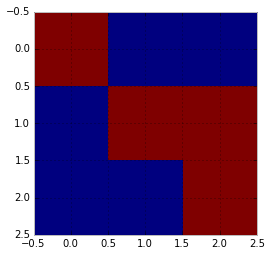Let's set up a General Linear Model using Matrix Algebra:

$y = \beta_1 x_1 + \beta_2 x_2$

$Y = X\beta + \eta$

$$\begin{bmatrix} 0.3\\ 0.4\\ 1.2\\ \end{bmatrix} = \begin{bmatrix} 1 & 0\\ 0 & 1\\ 1 & 1\\ \end{bmatrix} \begin{bmatrix} \beta_1 \beta_2 \end{bmatrix}$$
In :
Y = np.array([[0.3, 0.4, 0.7]]).T
print Y

X = np.array([[1, 0],
[0, 1],
[1, 1]])

print X

[[ 0.3]
[ 0.4]
[ 0.7]]
[[1 0]
[0 1]
[1 1]]


This can be solved using $\hat{\beta} = (X^T X)^{-1}X^TY$

In :
beta = np.linalg.pinv(X.T.dot(X)).dot(X.T).dot(Y)
beta

Out:
array([[ 0.3],
[ 0.4]])

Now let's set up an ANOVA-like GLM, where two variables do either occur or do not occur at random. We have a design matrix $X$ of size $\text{n_observations} \times \text{n_variables}$

In :
X = np.array([np.random.random(15) < .5, np.random.random(15) < .5]).T.astype(int)
X

Out:
array([[1, 1],
[0, 0],
[1, 0],
[1, 0],
[1, 0],
[1, 0],
[1, 0],
[1, 0],
[1, 1],
[0, 0],
[1, 0],
[0, 0],
[1, 1],
[0, 1],
[1, 1]])

Now set up the laten variables, we draw them from a standard normal distribution and make the mean of the first variable 1, that of the second variable 3

In :
Y = np.random.randn(15, 2)
Y[:, 0] += 1
Y[:, 1] += 3
Y

Out:
array([[ 0.13690094,  3.15445424],
[-1.23456025,  2.16547678],
[ 3.95299347,  3.28154208],
[-0.29761438,  1.70945387],
[-0.30799664,  1.92316424],
[ 0.46978728,  3.53511541],
[ 1.44381006,  4.20100229],
[ 1.70937268,  5.04859716],
[ 1.37540351,  4.02951691],
[ 2.46133296,  1.58895603],
[ 0.48321896,  3.95785619],
[ 0.78920216,  2.25337544],
[ 0.6497828 ,  2.82203775],
[-0.11051303,  4.45488919],
[ 1.3138075 ,  2.16161622]])

Now we multiply the latent variables with the design matrix, and sum over the columns so we get a 1D observation vector

In :
Y = np.sum(X * Y, 1)
beta = np.linalg.pinv(X.T.dot(X)).dot(X.T).dot(Y)
beta

Out:
array([ 0.89979026,  3.2998496 ])

The original parameters $\beta_1 = 1$ and $\beta_2$ = 3 are reconstructed with some precision...

Let's see what happens if we simulate data a 1000 times, so we get an idea of the sampling distribution of this 'experiment'. We add the found parameters to a list params. Note that the second parameter is very unlikely to occur (only 10% of observations).

In :
params = []
for i in np.arange(1000):
Y = np.random.randn(15, 2)
Y[:, 0] += 1
Y[:, 1] += 3
X = np.array([np.random.random(15) < .5, np.random.random(15) < .1]).T.astype(int)
Y = np.sum(X * Y, 1)
beta = np.linalg.pinv(X.T.dot(X)).dot(X.T).dot(Y)
params.append(beta)

In :
# cast list of numpy arrays to a 2D numpy array
params = np.array(params)

In :
plt.hist(params, bins=15, label=['a', 'b'])
plt.legend()

plt.axvline(1, color='k', lw=2)
plt.axvline(3, c='k', lw=2)

Out:
<matplotlib.lines.Line2D at 0x79257d0>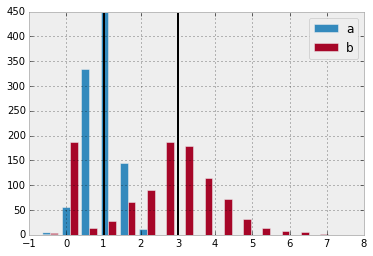The sampling distribution of variable b is much wider, because it occurs so rarely. Sometimes its even estimated zero, because it did not occur at all...

### Scipy¶

Now let's have a look at SciPy, a very powerful extension of numpy, containg state-of-the-art numerical algorithms.

A very nice one is the 'optimize'-module, which includes many standard optimizing algorithms

In :
from scipy import optimize


It is also a nice illustration of Python being a functional programming language: we can actually feed functions as objects to other functions. In the case of optimization this is really elegant: we can write a function to be optimized, and feed that to the optimizer. Here we have a function that returns the sum of squared errors of the variable $X$, fitted with $\beta$, where param1 is $\beta_1$ and param2 is $\beta_2$

In :
def obj_func(param1, param2):
params = np.array([param1, param2])
return np.sum((Y - X.dot(np.array(params)))**2)

In :
#Recall that param1 should be 1 and param 2 should be  3.
print obj_func(3, 3)
print obj_func(3, 10)
print obj_func(1, 3)

20.0883304674
100.676566839
5.43508779288


Now let's first do a grid search: we just try out lots of different parameter values and see what the objective function returns

In :
# Create simulated ata
X = np.array([np.random.random(15) < .5, np.random.random(15) < .5]).T.astype(int)
Y = np.random.randn(15, 2)
Y[:, 0] += 5
Y[:, 1] -= 2

Y = np.sum(X * Y, 1)

# Find OLS solution
beta = np.linalg.pinv(X.T.dot(X)).dot(X.T).dot(Y)
print beta

# Vectorize is a way of applying a function (in this case obj_func),
# to every element in a matrix
obj_func_vec = np.vectorize(obj_func)

# Set up the grid
x_lim = (-25, 25)
y_lim = (-25, 25)

# very IMPORTANT function. Can you see what it does?
x, y = np.meshgrid(np.arange(*x_lim), np.arange(*y_lim))
print x
print y

# Plot the result of the grid search
plt.figure(figsize=(12, 9))
plt.imshow(obj_func_vec(x, y), extent=(x_lim + y_lim), cmap=plt.cm.jet_r, origin='lower')
plt.grid(color='k')
plt.colorbar()
plt.xlabel('\beta_1')

# Put a point on the OLS-solution
plt.scatter(beta, beta, color='white')

[ 4.33100319 -3.02599482]
[[-25 -24 -23 ...,  22  23  24]
[-25 -24 -23 ...,  22  23  24]
[-25 -24 -23 ...,  22  23  24]
...,
[-25 -24 -23 ...,  22  23  24]
[-25 -24 -23 ...,  22  23  24]
[-25 -24 -23 ...,  22  23  24]]
[[-25 -25 -25 ..., -25 -25 -25]
[-24 -24 -24 ..., -24 -24 -24]
[-23 -23 -23 ..., -23 -23 -23]
...,
[ 22  22  22 ...,  22  22  22]
[ 23  23  23 ...,  23  23  23]
[ 24  24  24 ...,  24  24  24]]

Out:
<matplotlib.collections.PathCollection at 0x7cde0d0>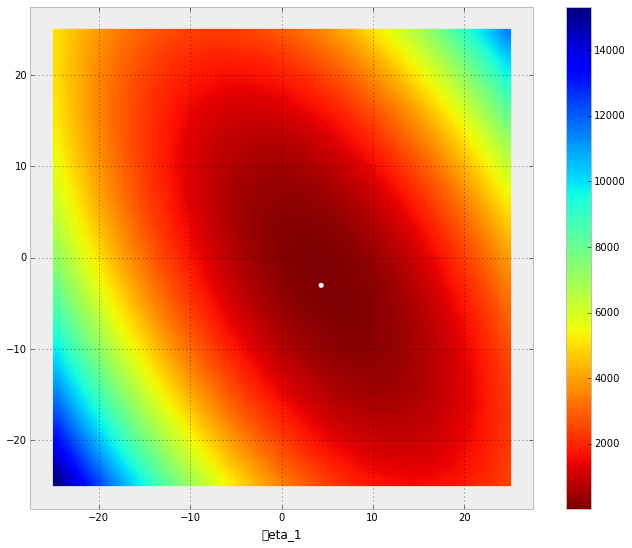As the OLS solution showed, the lowest values of the objective function are around the real values, $\beta_1 = 5$ and $\beta_2 = -2$.

In :
import scipy as sp
from scipy import optimize


Here we use a naive optimization algorithm, Nelder-Mead or Simplex. Note that many alternatives are available out-of-the-box.

In :
result = sp.optimize.minimize(lambda x: obj_func(*x), [0, 0], method='Nelder-Mead')
result

Out:
  status: 0
nfev: 151
success: True
fun: 18.217758186100077
x: array([ 4.33100973, -3.02601255])
message: 'Optimization terminated successfully.'
nit: 77

(The found parameters, 5.18 and -1.33 are very close to the real values, 5 and -2). Simplex found these in 75 iterations.

In :
print 'param 1: %.2f' % (result.x) # %.2f: Make a string of the variable x with 2-point precision
print 'param 2: %.2f' % (result.x)
print 'in %d iterations' % (result.nit) # %d: Make an integer-like string of result.nit

param 1: 4.33
param 2: -3.03
in 77 iterations


## Statsmodels¶

In analyzing real data one rarely wants to set up a design matrix using the plain linear algebra yourself. You want something like the R lm- and formula-functionality. Python can do this (almost) as well. For this one can use the statsmodels-library:

In :
import statsmodels.api as sm


This library can do a lot of the linear modeling one would usually do in R. It comes from econometrics and has a lot of models that are robust against autocorrelationa and heteroskedasticity. Unfortunately, support for hiearchial modeling is not that good yet (but we'll see a solution for that soon...)

So, let's fit the design matrix we just had:

In :
model = sm.OLS(Y, X)
fit = model.fit()
fit.summary()

/usr/local/lib/python2.7/dist-packages/scipy/stats/stats.py:1293: UserWarning: kurtosistest only valid for n>=20 ... continuing anyway, n=15
int(n))

Out:
Dep. Variable: R-squared: y 0.781 OLS 0.765 Least Squares 46.48 Mon, 03 Mar 2014 1.23e-05 17:38:04 -22.742 15 49.48 13 50.90 1
coef std err t P>|t| [95.0% Conf. Int.] 4.3310 0.492 8.811 0.000 3.269 5.393 -3.0260 0.659 -4.588 0.001 -4.451 -1.601
 Omnibus: Durbin-Watson: 2.594 1.223 0.273 0.894 -0.541 0.64 3.51 2.13

In real scenario's you usually a dataset in a csv-like format. You could use Python to do the parsing of this yourself:

In :
f = open('behavior_jasper.csv')

for line in f.readlines()[:10]: # Show first 10 lines of the file
print line

"id","trnr","stim","resp","resp2","resp3","resp4","RT","RT1","RT2","RT3","RT4","correct","movement","cue","jittertime1","jittertime2","cueonset","stimonset","subj","day","event","blockid","drug","prevcorr","NA","formercorrect"

"1",1,1,1,0,0,0,489.3,489.299999999999,0,0,0,1,-1,"SN",0,1500,10217.7,16468.7,"01","1",14,"S01D1B1",0,0,1,NA

"2",2,1,1,0,0,0,306.1,306.100000000002,0,0,0,1,-1,"SN",0,1500,20227.2,26478.2,"01","1",1,"S01D1B1",0,0,2,1

"3",3,1,1,0,0,0,360.2,360.200000000004,0,0,0,1,-1,"SN",1000,500,31223.1,36474.4,"01","1",1,"S01D1B1",0,0,3,1

"4",4,1,1,0,0,0,563.4,563.399999999994,0,0,0,1,-1,"AC",1500,0,41725.8,46470.6,"01","1",7,"S01D1B1",0,0,4,1

"5",5,1,1,0,0,0,395.6,395.599999999999,0,0,0,1,-1,"SN",500,1000,50722.4,56480.2,"01","1",3,"S01D1B1",0,0,5,1

"6",6,2,2,0,0,0,470.2,470.199999999997,0,0,0,1,1,"AC",1500,0,61718.2,66476.4,"01","1",7,"S01D1B1",0,0,6,1

"7",7,2,2,0,0,0,509,509,0,0,0,1,1,"AC",500,1000,70728.1,76472.6,"01","1",9,"S01D1B1",0,0,7,1

"8",8,1,1,0,0,0,549.4,549.400000000009,0,0,0,1,-1,"AC",1500,0,81724,86468.8,"01","1",9,"S01D1B1",0,0,8,1

"9",9,1,1,0,0,0,380,380,0,0,0,1,-1,"SN",500,1000,90720.6,96478.4,"01","1",3,"S01D1B1",0,0,9,1



You would probably use something like the split()-attribute of string objects

In :
'Ik,ben,Gilles'.split(',')

Out:
['Ik', 'ben', 'Gilles']
In :
f = open('/home/gdholla1/notebooks_demo/behavior_jasper.csv')

d = {}

line = line.split(',')
#print line

print keys

"id","trnr","stim","resp","resp2","resp3","resp4","RT","RT1","RT2","RT3","RT4","correct","movement","cue","jittertime1","jittertime2","cueonset","stimonset","subj","day","event","blockid","drug","prevcorr","NA","formercorrect"



But let me tell you: if you find yourself writing this kind of 'boiler plate code', you're doing something wrong: someone already wrote this, and probably did a better job than you can do. Especially as he usually did it in the whole open source-github-model, making rigorous testing and code reviewing possible.

## Pandas¶

Pandas is an excellent choice as a library to read and prepare data for analysis. It implements a DataFrame-object, which is very similar to the dataframe-object in R.

In :
import pandas

data

Out:
<class 'pandas.core.frame.DataFrame'>
Int64Index: 8399 entries, 0 to 8398
Data columns (total 27 columns):
id               8399  non-null values
trnr             8399  non-null values
stim             8399  non-null values
resp             8399  non-null values
resp2            8399  non-null values
resp3            8399  non-null values
resp4            8399  non-null values
RT               8399  non-null values
RT1              8399  non-null values
RT2              8399  non-null values
RT3              8399  non-null values
RT4              8399  non-null values
correct          8399  non-null values
movement         8393  non-null values
cue              8399  non-null values
jittertime1      8399  non-null values
jittertime2      8399  non-null values
cueonset         8020  non-null values
stimonset        8000  non-null values
subj             8399  non-null values
day              8399  non-null values
event            8006  non-null values
blockid          8399  non-null values
drug             8399  non-null values
prevcorr         8399  non-null values
NA               8399  non-null values
formercorrect    8319  non-null values
dtypes: float64(8), int64(17), object(2)

In :
data.subj.unique()

Out:
array([ 1,  2,  3,  4,  5,  6,  7,  8,  9, 10, 11, 12, 13, 14, 15, 16, 17,
18, 19, 20])
In :
###Groupby()


A very handy function of the DataFrame-class is groupby(): it fits very well in the split-apply-combine (see everywehere) line of thought. Here we get the mean RT of every individual subject, in one line of code:

In :
data.groupby('subj').mean()['RT']

Out:
subj
1       424.617143
2       395.185476
3       437.465952
4       418.970238
5       442.447143
6       533.706190
7       609.438663
8       435.460000
9       548.614286
10      349.241429
11      466.269048
12      427.157381
13      376.915238
14      450.543333
15      512.585952
16      584.598571
17      406.131667
18      577.838095
19      385.023571
20      444.374762
Name: RT, dtype: float64

And we plot a histogram of the per-subject-mean-RTs...

In :
data.groupby('subj').mean()['RT'].hist()

Out:
<matplotlib.axes.AxesSubplot at 0x830ca10>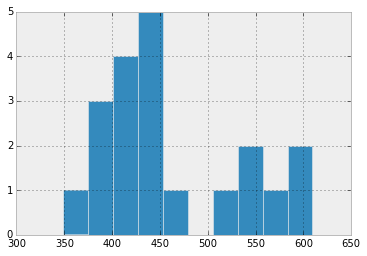In :
data.groupby(['drug', 'correct']).mean().RT

Out:
drug  correct
0     0          466.732226
1          498.216629
99           0.000000
1     0          442.085297
1          488.707662
99           0.000000
Name: RT, dtype: float64

Here we see the functional programming again: we can create a very simple standard-error function using the lambda'-operator:

In :
se = lambda x: np.std(x) / np.sqrt(len(x)) # Take the standard deviation of the dataframe and divide it
# by the squareroot of the number of elements in the dataframe


Now apply this to every group in the groupby-object.

In :
data.groupby(['drug', 'correct']).RT.agg({'m':np.mean, 'se':se})

Out:
m se
drug correct
0 0 466.732226 9.590618
1 498.216629 3.591182
99 0.000000 0.000000
1 0 442.085297 8.577553
1 488.707662 3.440124
99 0.000000 0.000000

The 'groupby' function actually return an object (of the class DataFrameGroupBy) with some very nice properties.

In :
data.groupby('subj')

Out:
<pandas.core.groupby.DataFrameGroupBy object at 0x8519a90>

For example, we can iterate through it, at every iteration we then have a grouping key/label (g) of this group, as well as a dataframe of all rows belonging to this grouping.

In :
for g, d in data.groupby('subj'):
print g, d.RT.mean()

1 424.617142857
2 395.18547619
3 437.465952381
4 418.970238095
5 442.447142857
6 533.706190476
7 609.438663484
8 435.46
9 548.614285714
10 349.241428571
11 466.269047619
12 427.157380952
13 376.915238095
14 450.543333333
15 512.585952381
16 584.598571429
17 406.131666667
18 577.838095238
19 385.023571429
20 444.374761905


Using groupby, it's really easy to plot RT distributions for every single subject

In :
plt.figure(figsize=(16, 20))

for g, d in data.groupby('subj'):    # Iterate over all groupings, g contains the group label, d the dataframe.
plt.subplot(5,4,g)               # Make a subplot of 5 rows and 4 columns, and plot the next thing in plot number g
plt.hist(d.RT)                   # Plot histogram of RTs
plt.xlim(0, 2000)                # limit x-axis from 0 to 2000 miliseconds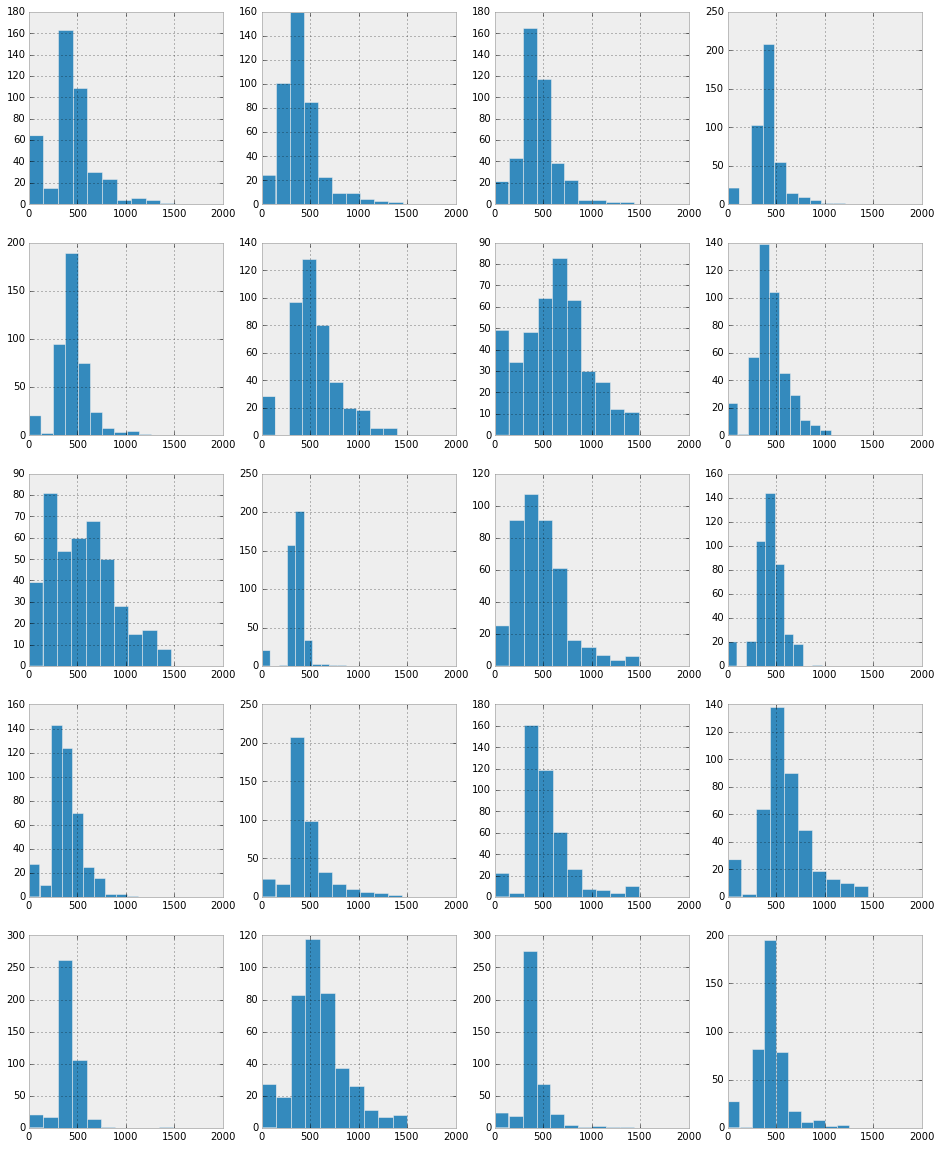We can also combine groupby's. For example, in this dataset, we have a 'speed' and 'accurate'-condition. Let's group the first grouping again on this 'cue'-variable within the same plot:

In :
plt.figure(figsize=(20, 35))

for g, d in data.groupby('subj'): # First group on subject...

plt.subplot(7,3,g)

for drug, d2 in d.groupby('cue'): # Then on cue...
plt.hist(d2.RT, label=str(drug), bins=np.arange(0, 2000, 50), alpha=0.6)   # All these histogram are all in the same subplot
# The alpha-parameter makes the histograms
# slightly transparent
plt.xlim(0, 2000)

plt.title('Subjec %s' % g)
plt.legend()
plt.savefig('RT_histograms.pdf')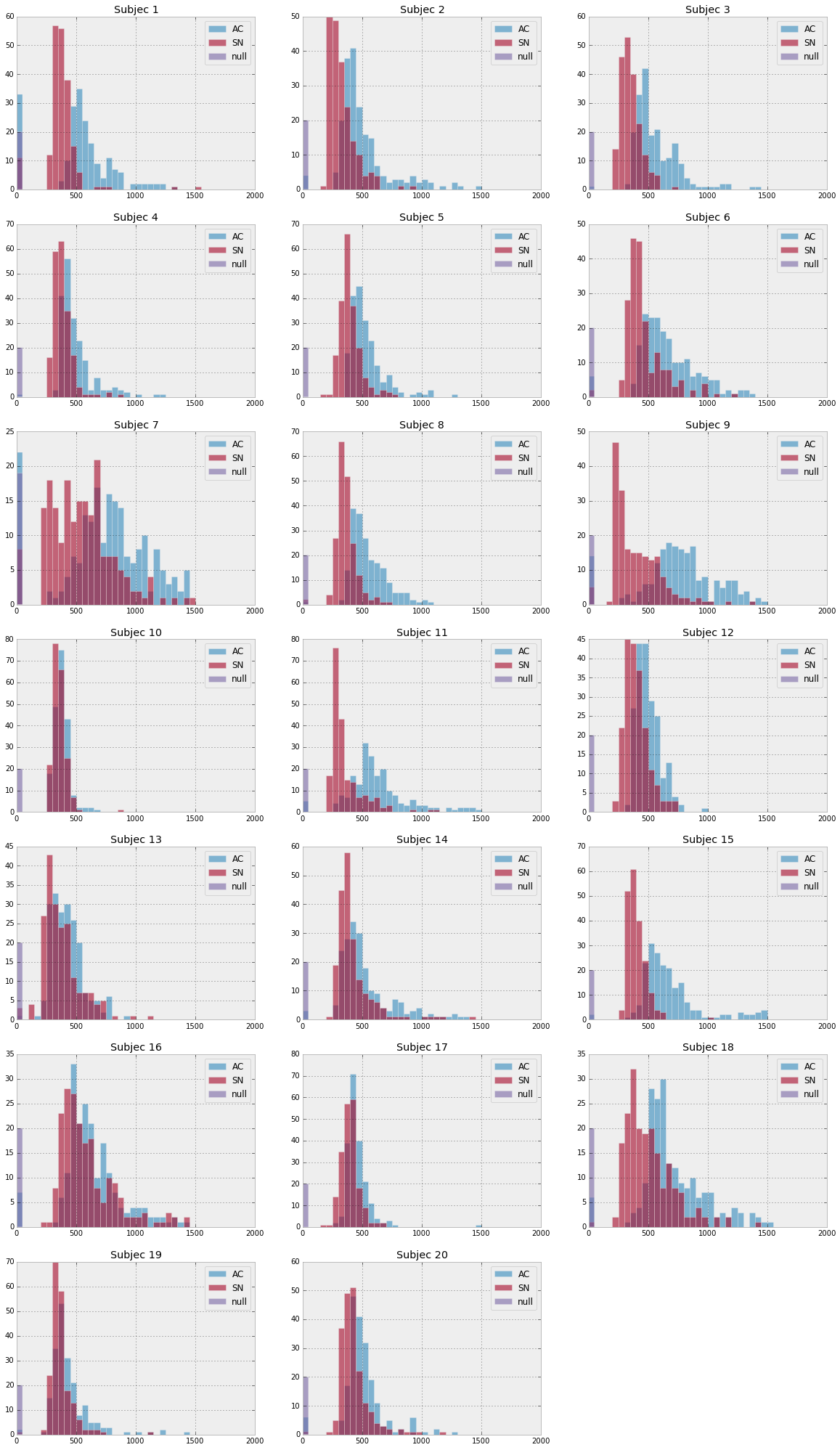In :
# If we want to do traditional psychology-like ANOVA's, we often want to average
# over all subject-condition combinations. Here we create a new dataframe 'd',
# that contains such a dataset

d = data.groupby(['subj', 'cue', 'day'], as_index=False).mean()

d[['subj', 'RT', 'correct', 'day', 'cue']].head(25) # Here we say that we only want to see a limited
# number of columns, namely subj, RT, correct, day
# and cue.

Out:
subj RT correct day cue
0 1 553.242 0.85 1 AC
1 1 488.837 0.73 2 AC
2 1 382.056 0.88 1 SN
3 1 359.257 0.82 2 SN
4 1 0.000 99.00 1 null
5 1 0.000 99.00 2 null
6 2 511.382 0.41 1 AC
7 2 490.547 0.47 2 AC
8 2 334.079 0.54 1 SN
9 2 323.771 0.44 2 SN
10 2 0.000 99.00 1 null
11 2 0.000 99.00 2 null
12 3 612.855 0.85 1 AC
13 3 521.103 0.78 2 AC
14 3 371.893 0.72 1 SN
15 3 331.506 0.58 2 SN
16 3 0.000 99.00 1 null
17 3 0.000 99.00 2 null
18 4 467.012 0.96 1 AC
19 4 525.962 0.94 2 AC
20 4 377.138 0.87 1 SN
21 4 389.563 0.86 2 SN
22 4 0.000 99.00 1 null
23 4 0.000 99.00 2 null
24 5 549.325 0.97 1 AC
In :
# Now we can have statistics on these averaged-over data
d.groupby(['cue', 'drug']).agg([np.mean, lambda x: x.std() / np.sqrt(len(x))]).RT

Out:
mean <lambda>
cue drug
AC 0 561.68780 28.496958
1 556.17440 22.868897
SN 0 420.53025 19.540533
1 398.88550 12.372063
null 0 0.00000 0.000000
1 0.00000 0.000000

Statsmodels has very nice 'formula'-api, where we can set up GLMs using to easy-to-read formula's, as in R:

In :
import statsmodels.formula.api as smf

In :
model = smf.ols('RT ~ subj + C(cue)*C(drug)', d[d.cue != 'null'])

r = model.fit()

In :
r.summary()

Out:
Dep. Variable: R-squared: RT 0.083 OLS 0.076 Least Squares 11.95 Mon, 03 Mar 2014 1.66e-07 17:48:23 -2566.4 400 5141. 396 5157. 3
coef std err t P>|t| [95.0% Conf. Int.] 1.2334 0.037 33.259 0.000 1.160 1.306 -99.5550 21.031 -4.734 0.000 -140.901 -58.209 16.9590 21.031 0.806 0.420 -24.387 58.305 53.2100 29.742 1.789 0.074 -5.262 111.682 24.6678 0.742 33.259 0.000 23.210 26.126
 Omnibus: Durbin-Watson: 122.776 2.094 0 792.509 1.136 8.11e-173 9.511 nan

Using anova_lm, we can transform the GLM/OLS-interpretation to a (more familiar) ANOVA-table:

In :
from statsmodels.stats.anova import anova_lm
anova_lm(r)

Out:
df sum_sq mean_sq F PR(>F)
C(cue) 1 532170.250000 532170.250000 24.064494 0.000001
C(drug) 1 189782.209600 189782.209600 8.581864 0.003592
C(cue):C(drug) 1 70782.602500 70782.602500 3.200757 0.074368
subj 1 9922.212445 9922.212445 0.448678 0.503354
Residual 396 8757276.201000 22114.333841 NaN NaN
In :
### Integration with R


Still, to be honest, the statistical libraries of R, especially in the domains of clustering and random mixed effects models, are still better than in Python. Still, getting data from one environment to the other and back can be a bit of a hassle. No worries: the rmagic plugin of IPython does a great job in running R wihtin your IPython notebook.

In :
%load_ext rmagic


Let's fit a linear mixed effects models with levels for subjects and days. First we remove the trials with a null 'cue':

In :
d = data[data.cue != 'null']


Note that we can easily insert the Python DataFrame-variable d into R using the %%R -i INPUT_VARIABLE and export some stuff again using the %%R -o OUTPUT_VARIABLE trick:

In :
%%R -i d -o model
library(lmerTest)
model = lmer('RT ~ cue*drug + (1|subj) + (1|day)', d)

print(summary(model))

print('*************')

print(anova(model))

library(languageR)
#plotLMER.fnc(model)
plotLMER.fnc(model)

Linear mixed model fit by REML ['merModLmerTest']
Formula: RT ~ cue * drug + (1 | subj) + (1 | day)
Data: d

REML criterion at convergence: 106688.3

Random effects:
Groups   Name        Variance Std.Dev.
subj     (Intercept)  6005     77.49
day      (Intercept)     0      0.00
Residual             35991    189.71
Number of obs: 8000, groups: subj, 20; day, 2

Fixed effects:
Estimate Std. Error       df t value Pr(>|t|)
(Intercept)  561.688     17.839   21.000  31.487   <2e-16 ***
cueSN       -141.158      5.999 7977.000 -23.529   <2e-16 ***
drug          -5.513      5.999 7977.000  -0.919   0.3581
cueSN:drug   -16.131      8.484 7977.000  -1.901   0.0573 .
---
Signif. codes:  0 ‘***’ 0.001 ‘**’ 0.01 ‘*’ 0.05 ‘.’ 0.1 ‘ ’ 1

Correlation of Fixed Effects:
(Intr) cueSN  drug
cueSN      -0.168
drug       -0.168  0.500
cueSN:drug  0.119 -0.707 -0.707
 "*************"
Analysis of Variance Table of type 3  with  Satterthwaite
approximation for degrees of freedom
Sum Sq  Mean Sq NumDF DenDF F.value    Pr(>F)
cue      44535142 44535142     1  7977  553.63 < 2.2e-16 ***
drug       368783   368783     1  7977   10.25  0.001375 **
cue:drug   130110   130110     1  7977    3.62  0.057293 .
---
Signif. codes:  0 ‘***’ 0.001 ‘**’ 0.01 ‘*’ 0.05 ‘.’ 0.1 ‘ ’ 1
effect size (range) for  cue is  141.1576
effect size (range) for  drug is  5.5134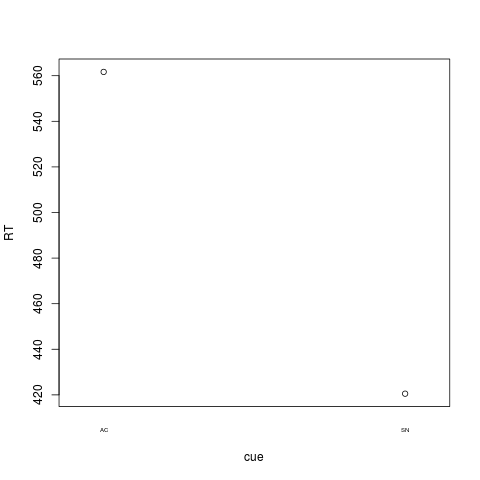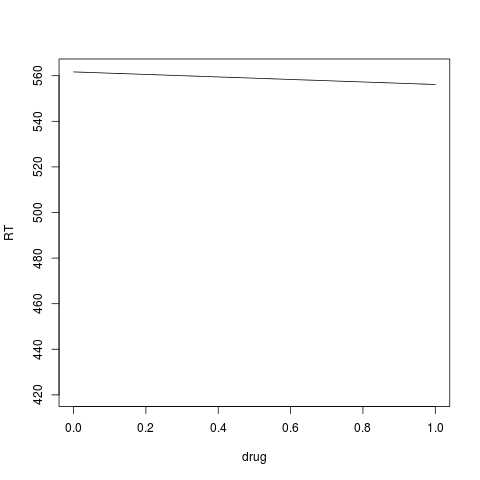## Hierachial Drift Diffusion Model¶

One very nice Python library for cognitive neuroscientists is the Hiearchial Drift Diffusion Model of Thomas Wiecki and Imre Sofer. It fits the Drift Diffusion Model in a Bayesian framework, using Markov Chain Monte Carlo-sampling, as implemented by the also-really-great-library pymc.

We can fit our data in only a few lines of code:

In :
import hddm


Note that you can get documentation of a library/function/object using a question mark:

In :
hddm.HDDM?

In :
# Set up some extra variables

data['rt'] = data.RT / 1000.       # RTs in miliseconds
data['response'] = data.correct    # We model responses as correct vs incorrect
data['subj_idx'] = data['subj']    # For hiearchial modeling the subjects have to be indicated with a subj_idx-column

d = data[(data.resp != 0) & (data.resp != 99)].copy() # Remove null-events and misses


Let's try out a model where the threshold depends on which cue the subject got and the drift rate can differ over different sessions (first versus second session...)

In :
model = hddm.HDDM(d[['rt', 'subj_idx', 'response', 'cue', 'day']], depends_on={'a':'cue', 'v':'day'}, include=('p_outlier'))

In :
model.sample(1000, 20)

 [-----------------100%-----------------] 1000 of 1000 complete in 221.0 sec
Out:
<pymc.MCMC.MCMC at 0x10ee2410>

Now we can show some basic stats, plot posteriors to chec for convergence, etc:

In :
model.print_stats()

                   mean       std      2.5q       25q       50q       75q     97.5q    mc err
a(AC)          1.463675  0.077425  1.339998  1.413952  1.458223  1.501251  1.651733  0.005227
a(SN)          0.947748  0.072160  0.842701  0.904862  0.937567  0.980855  1.113020  0.005039
a_std          0.263406  0.073476  0.195297  0.227958  0.249199  0.275773  0.483889  0.006813
a_subj(AC).1   1.994009  0.168938  1.760144  1.894681  1.972304  2.061612  2.353467  0.014013
a_subj(AC).2   1.211353  0.034994  1.149247  1.186546  1.210194  1.236210  1.284121  0.001308
a_subj(AC).3   1.412392  0.050743  1.313467  1.376233  1.412840  1.448187  1.515622  0.001921
a_subj(AC).4   1.446388  0.061193  1.328372  1.405283  1.444160  1.488635  1.568823  0.002801
a_subj(AC).5   1.834938  0.441802  1.607680  1.700015  1.751451  1.806938  3.718390  0.045056
a_subj(AC).6   1.527825  0.054995  1.423842  1.488719  1.524494  1.566683  1.642083  0.001997
a_subj(AC).7   1.715825  0.053029  1.608993  1.682150  1.717570  1.751977  1.814966  0.001791
a_subj(AC).8   1.672402  0.073494  1.536535  1.624128  1.672269  1.723643  1.812753  0.003889
a_subj(AC).9   1.777783  0.061424  1.655669  1.734781  1.778510  1.822878  1.896954  0.002224
a_subj(AC).10  1.066542  0.232128  0.930838  0.985618  1.015337  1.052520  2.097272  0.023698
a_subj(AC).11  1.424235  0.045006  1.341975  1.391336  1.422399  1.457579  1.510049  0.001557
a_subj(AC).12  1.648754  0.296319  1.473685  1.558639  1.604593  1.649093  3.001803  0.030020
a_subj(AC).13  1.020951  0.032094  0.957238  1.000315  1.020469  1.041186  1.084331  0.001102
a_subj(AC).14  1.176035  0.037139  1.106591  1.149601  1.174742  1.203191  1.249022  0.001305
a_subj(AC).15  2.024677  0.092389  1.854176  1.962860  2.022468  2.087399  2.226256  0.004681
a_subj(AC).16  1.382529  0.049836  1.281250  1.349247  1.381970  1.415308  1.483671  0.001872
a_subj(AC).17  1.135750  0.043870  1.057191  1.104089  1.136151  1.163248  1.226909  0.001711
a_subj(AC).18  1.719998  0.058868  1.603240  1.681157  1.719370  1.759297  1.837382  0.002480
a_subj(AC).19  1.118235  0.043427  1.037311  1.091107  1.114866  1.146646  1.209332  0.002153
a_subj(AC).20  1.456656  0.075539  1.321757  1.403450  1.451706  1.503893  1.622788  0.004725
a_subj(SN).1   0.808141  0.047368  0.732026  0.778084  0.803931  0.832635  0.907761  0.003121
a_subj(SN).2   0.740186  0.024007  0.694647  0.724350  0.738706  0.755647  0.790493  0.001134
a_subj(SN).3   0.750961  0.026365  0.701369  0.732738  0.751085  0.768030  0.804197  0.001288
a_subj(SN).4   0.815342  0.033238  0.753557  0.792089  0.814606  0.838713  0.876802  0.001728
a_subj(SN).5   0.992253  0.339406  0.855282  0.901876  0.928628  0.956385  2.470501  0.035029
a_subj(SN).6   0.945481  0.031728  0.887602  0.924079  0.944643  0.966488  1.010432  0.001307
a_subj(SN).7   1.212280  0.037989  1.138787  1.186440  1.213327  1.238712  1.283199  0.001555
a_subj(SN).8   0.754748  0.025344  0.709914  0.737195  0.753541  0.770882  0.804546  0.001038
a_subj(SN).9   0.959737  0.031234  0.901623  0.939174  0.958364  0.980815  1.022157  0.001442
a_subj(SN).10  0.905864  0.215236  0.778363  0.833113  0.860566  0.892471  1.872019  0.022031
a_subj(SN).11  0.750864  0.026466  0.700679  0.732439  0.748804  0.768758  0.804120  0.001306
a_subj(SN).12  0.976545  0.223242  0.880004  0.915999  0.940152  0.963757  1.980672  0.022835
a_subj(SN).13  0.883309  0.029405  0.829996  0.863299  0.881837  0.901539  0.948233  0.001242
a_subj(SN).14  0.900580  0.030431  0.845246  0.879060  0.900113  0.921373  0.960084  0.001350
a_subj(SN).15  0.771097  0.029933  0.718279  0.749553  0.770854  0.789449  0.834980  0.001339
a_subj(SN).16  1.171650  0.040283  1.097070  1.143970  1.170431  1.198708  1.254567  0.001789
a_subj(SN).17  0.805598  0.034908  0.741697  0.781147  0.804175  0.827507  0.879179  0.002014
a_subj(SN).18  1.107053  0.036847  1.038261  1.080884  1.106275  1.131478  1.179768  0.001712
a_subj(SN).19  0.847983  0.036043  0.782036  0.822863  0.846012  0.871522  0.923186  0.001825
a_subj(SN).20  0.949983  0.050376  0.862748  0.914477  0.945636  0.980855  1.058617  0.003320
v(1)           1.806330  0.272549  1.303230  1.625199  1.798791  1.977760  2.358636  0.010485
v(2)           1.610263  0.284228  1.086057  1.422166  1.595899  1.786295  2.231234  0.012380
v_std          1.190262  0.210165  0.921162  1.060066  1.149684  1.268614  1.775905  0.016348
v_subj(1).1    2.765472  0.271765  2.297778  2.592676  2.744274  2.905645  3.274687  0.020058
v_subj(1).2   -0.095202  0.153541 -0.400847 -0.195830 -0.095392  0.000771  0.204325  0.004990
v_subj(1).3    1.248227  0.147489  0.964059  1.145837  1.247517  1.354058  1.521810  0.004898
v_subj(1).4    3.068625  0.208558  2.637131  2.932705  3.060667  3.221674  3.479382  0.009585
v_subj(1).5    2.587316  0.609315  2.127705  2.357664  2.476594  2.603347  5.334478  0.060838
v_subj(1).6    1.307968  0.143521  1.029825  1.205817  1.314188  1.411205  1.583063  0.005207
v_subj(1).7   -0.036447  0.096151 -0.226175 -0.101412 -0.030596  0.027753  0.151806  0.003242
v_subj(1).8    2.230392  0.174022  1.893507  2.114408  2.226958  2.341649  2.577380  0.007765
v_subj(1).9    0.573117  0.108341  0.366484  0.500267  0.573308  0.652115  0.783110  0.003882
v_subj(1).10   3.851311  0.570754  3.291743  3.585972  3.741271  3.923708  6.189390  0.054915
v_subj(1).11   0.843076  0.126353  0.594319  0.760946  0.848113  0.931066  1.088890  0.003835
v_subj(1).12   2.264837  0.441672  1.879092  2.080445  2.195397  2.321148  4.287334  0.042589
v_subj(1).13   0.220017  0.147810 -0.054477  0.127604  0.213033  0.317841  0.529931  0.004811
v_subj(1).14   1.166737  0.159107  0.856253  1.059974  1.171513  1.271863  1.485750  0.005855
v_subj(1).15   2.988814  0.182953  2.645796  2.860447  2.983818  3.114185  3.359821  0.008800
v_subj(1).16   1.302350  0.129645  1.038658  1.214928  1.304691  1.382603  1.564615  0.005003
v_subj(1).17   2.348589  0.194195  1.969944  2.219121  2.341064  2.475918  2.745961  0.006200
v_subj(1).18   1.148917  0.124792  0.911924  1.067964  1.139330  1.233830  1.402614  0.004536
v_subj(1).19   2.978042  0.210027  2.544833  2.840526  2.987470  3.123830  3.369391  0.008560
v_subj(1).20   3.056704  0.230889  2.606082  2.898574  3.048550  3.208592  3.527064  0.011127
v_subj(2).1    2.763453  0.328124  2.267005  2.556600  2.728920  2.928726  3.486977  0.025564
v_subj(2).2   -0.136849  0.147012 -0.438952 -0.234061 -0.135150 -0.034025  0.147735  0.004887
v_subj(2).3    1.167631  0.157340  0.861351  1.061099  1.164332  1.265655  1.486939  0.006437
v_subj(2).4    2.457412  0.191531  2.066620  2.315692  2.468671  2.583196  2.833930  0.007433
v_subj(2).5    2.900152  0.651827  2.418691  2.645358  2.787679  2.920662  5.784336  0.064853
v_subj(2).6    1.298229  0.118369  1.065487  1.217369  1.300176  1.380751  1.525682  0.003905
v_subj(2).7   -0.056072  0.108432 -0.271093 -0.127178 -0.055098  0.016340  0.151681  0.003786
v_subj(2).8    2.210578  0.174702  1.879052  2.094989  2.208694  2.326719  2.558938  0.007837
v_subj(2).9    0.601789  0.113290  0.377548  0.524422  0.600700  0.678088  0.817635  0.003632
v_subj(2).10   4.023343  0.708608  3.358051  3.712290  3.888125  4.080516  6.963064  0.069079
v_subj(2).11   0.612790  0.150734  0.341421  0.500934  0.616212  0.719122  0.910660  0.004984
v_subj(2).12   2.735828  0.510868  2.301992  2.523191  2.666135  2.783133  5.185865  0.050186
v_subj(2).13   0.358551  0.161650  0.036945  0.251750  0.355812  0.461888  0.679638  0.005243
v_subj(2).14   0.659922  0.142605  0.387744  0.560090  0.664367  0.756284  0.930886  0.004707
v_subj(2).15   2.009412  0.156588  1.695465  1.904082  2.004381  2.114354  2.318553  0.006331
v_subj(2).16   0.959517  0.127753  0.709275  0.870224  0.954486  1.042783  1.209519  0.004339
v_subj(2).17   1.890387  0.169918  1.557287  1.773781  1.891568  2.013283  2.222556  0.006479
v_subj(2).18   1.000125  0.109785  0.781323  0.923528  1.003185  1.077723  1.202660  0.003815
v_subj(2).19   2.268007  0.176778  1.921648  2.150749  2.275983  2.393385  2.599028  0.006741
v_subj(2).20   2.261345  0.177685  1.908375  2.145089  2.259054  2.382962  2.610736  0.007505
t              0.240808  0.009497  0.221680  0.234661  0.240512  0.247337  0.259937  0.000359
t_std          0.040044  0.007303  0.028361  0.034916  0.039190  0.044387  0.057002  0.000289
t_subj.1       0.271061  0.004233  0.260984  0.269753  0.272025  0.273815  0.276128  0.000249
t_subj.2       0.192391  0.002675  0.187583  0.190516  0.192136  0.193984  0.198228  0.000131
t_subj.3       0.225428  0.003315  0.217998  0.223947  0.225880  0.227517  0.231343  0.000162
t_subj.4       0.265190  0.002428  0.259493  0.263784  0.265458  0.266901  0.269199  0.000138
t_subj.5       0.243259  0.014049  0.184591  0.244067  0.245824  0.247568  0.250575  0.001436
t_subj.6       0.272572  0.003075  0.265986  0.270789  0.272762  0.274690  0.277926  0.000137
t_subj.7       0.178758  0.005335  0.167849  0.175211  0.179254  0.182481  0.188522  0.000202
t_subj.8       0.247189  0.001817  0.243439  0.246010  0.247241  0.248439  0.250638  0.000086
t_subj.9       0.170972  0.003927  0.162142  0.168729  0.171343  0.173546  0.178183  0.000156
t_subj.10      0.255891  0.008719  0.218606  0.256126  0.257774  0.259135  0.262009  0.000881
t_subj.11      0.216166  0.003259  0.209426  0.214808  0.216415  0.217970  0.221973  0.000172
t_subj.12      0.232627  0.009264  0.193638  0.232174  0.233936  0.235630  0.240821  0.000913
t_subj.13      0.188558  0.003480  0.181853  0.185868  0.188815  0.191243  0.194636  0.000153
t_subj.14      0.233505  0.002563  0.228331  0.231711  0.233678  0.235312  0.238370  0.000116
t_subj.15      0.284809  0.002378  0.279646  0.283387  0.285103  0.286472  0.288735  0.000114
t_subj.16      0.291847  0.005478  0.279729  0.288749  0.292651  0.295618  0.300916  0.000239
t_subj.17      0.269264  0.004870  0.256832  0.267518  0.269920  0.271833  0.277640  0.000280
t_subj.18      0.226887  0.005541  0.209822  0.224741  0.227969  0.230424  0.234596  0.000280
t_subj.19      0.248362  0.003090  0.241501  0.246573  0.248721  0.250471  0.253360  0.000156
t_subj.20      0.276832  0.009876  0.256914  0.273111  0.279997  0.283793  0.289554  0.000799
p_outlier      0.010414  0.003500  0.007207  0.008795  0.009800  0.010898  0.024310  0.000335
DIC: -2980.763592
deviance: -3093.684019
pD: 112.920427

In :
model.plot_posteriors()

Plotting a(AC)
Plotting a(SN)
Plotting a_std
Plotting v(1)
Plotting v(2)
Plotting v_std
Plotting t
Plotting t_std
Plotting p_outlier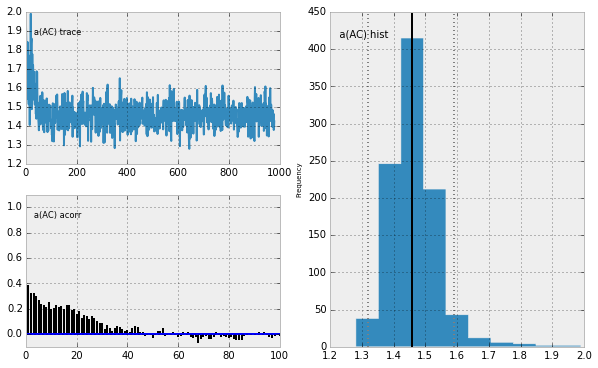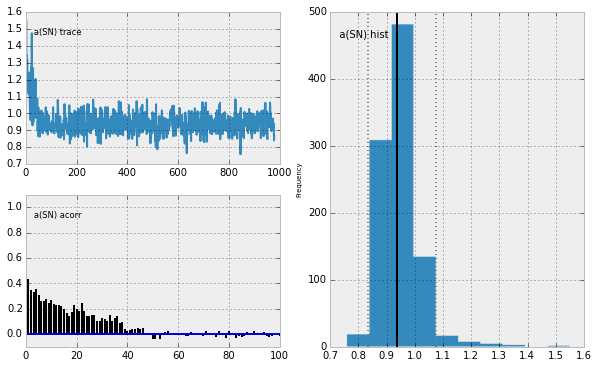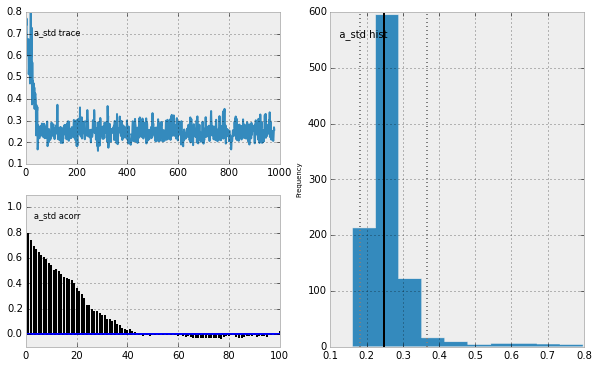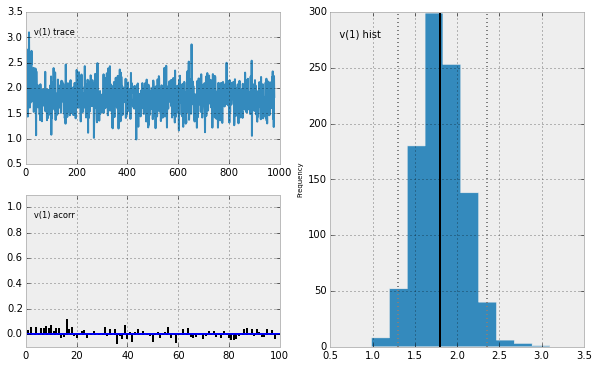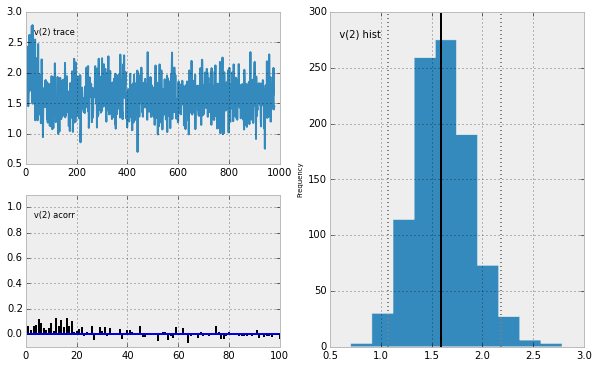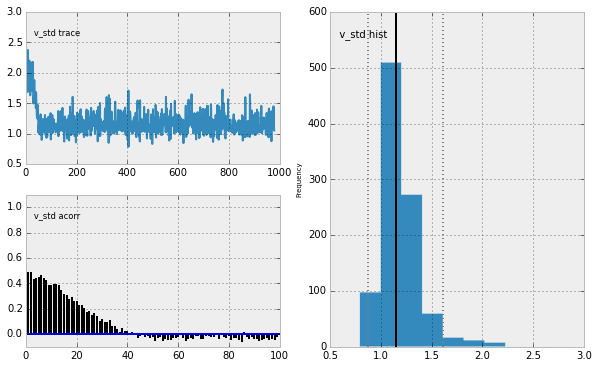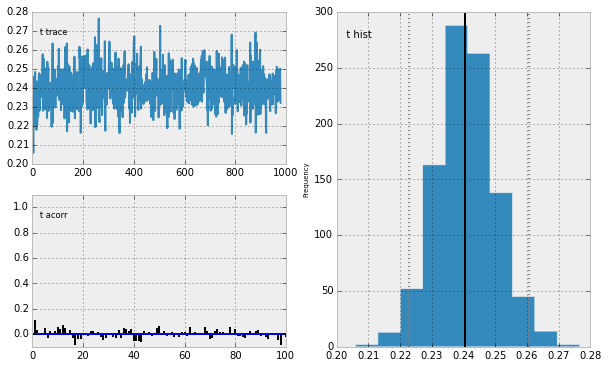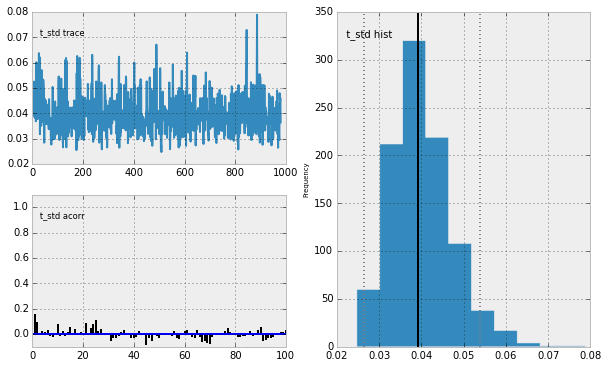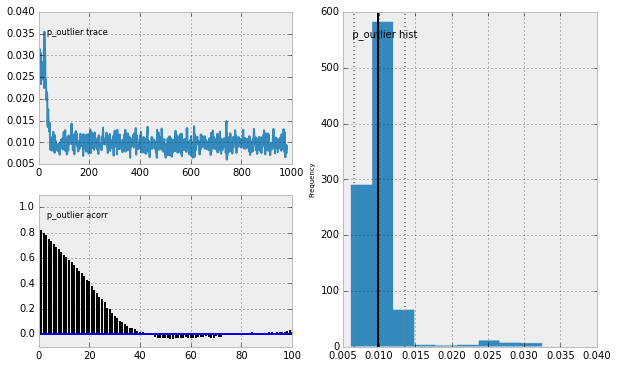# Nibabel¶

If you're doing neuroimaing, both structural and functional, nibabel is essential: it's a way of loading in MR data from nifti-, but also other files.

In :
import nibabel as nb


Here we import some QSM-data

In :
image = nb.load('/home/gdholla1/ERC_data/QSM_data/Young/AD2T_QSM.nii')


Note that the image-variable only contains some pointers to the file, header and affine info:

In :
print image

<class 'nibabel.nifti1.Nifti1Image'>
data shape (312, 56, 384)
affine:
[[  4.99999315e-01  -9.46268789e-04   2.76133796e-04  -7.82130051e+01]
[ -8.35519459e-04  -5.66280544e-01   1.65233091e-01   1.62180691e+01]
[ -2.38080169e-08   1.98276758e-01   4.71908838e-01  -6.86508713e+01]
[  0.00000000e+00   0.00000000e+00   0.00000000e+00   1.00000000e+00]]
sizeof_hdr      : 348
data_type       :
db_name         :
extents         : 0
session_error   : 0
regular         : r
dim_info        : 57
dim             : [  3 312  56 384   1   1   1   1]
intent_p1       : 0.0
intent_p2       : 0.0
intent_p3       : 0.0
intent_code     : none
datatype        : float32
bitpix          : 32
slice_start     : 0
pixdim          : [-1.          0.5         0.59999019  0.5         0.043       0.          0.
0.        ]
vox_offset      : 352.0
scl_slope       : 1.0
scl_inter       : 0.0
slice_end       : 0
slice_code      : unknown
xyzt_units      : 10
cal_max         : 0.687585234642
cal_min         : -0.535814523697
slice_duration  : 0.0
toffset         : 0.0
glmax           : 0
glmin           : 0
descrip         : FSL4.1
aux_file        :
qform_code      : scanner
sform_code      : scanner
quatern_b       : 0.985854029655
quatern_c       : -0.000823696784209
quatern_d       : -0.000140060074045
qoffset_x       : -78.2130050659
qoffset_y       : 16.2180690765
qoffset_z       : -68.6508712769
srow_x          : [  4.99999315e-01  -9.46268789e-04   2.76133796e-04  -7.82130051e+01]
srow_y          : [ -8.35519459e-04  -5.66280544e-01   1.65233091e-01   1.62180691e+01]
srow_z          : [ -2.38080169e-08   1.98276758e-01   4.71908838e-01  -6.86508713e+01]
intent_name     : Unknown
magic           : n+1

In :
print image.shape # Shape of the image

(312, 56, 384)


This is really nice: what is the orientation of the data? Here we see that as X (first dimension) goes up, we go to the right, as y goes up, we go to the back (posterior) and as Z goes up, we go up (superior).

In :
nb.orientations.aff2axcodes(image.get_affine())

Out:
('R', 'P', 'S')

We can get the actual data in the nifti-file using get_data():

In :
data = image.get_data()

In :
type(data), data.shape

Out:
(numpy.core.memmap.memmap, (312, 56, 384))

Now we can also use imshow to show the data:

In :
plt.figure(figsize=(14, 10))
plt.imshow(data[:, 27, 100:325].T, cmap=plt.cm.gray, origin='lower') # T transforms the data, so that the rows of the matrix
# correspond to the y-axis and the columns to the x-axis
# origin='lower' makes sure both axis go 'up'.

Out:
<matplotlib.image.AxesImage at 0xce5afd0>`